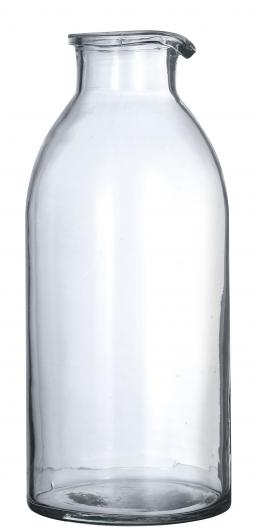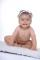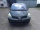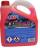# The shop

The shop has 3 hectoliters of water. How many liter bottles is it?

Correct result:

n =  300

#### Solution:We would be pleased if you find an error in the word problem, spelling mistakes, or inaccuracies and send it to us. Thank you!Tips to related online calculators
Do you know the volume and unit volume, and want to convert volume units?

## Next similar math problems:

• Here isHere is a data set (n=117) that has been sorted. 10.4 12.2 14.3 15.3 17.1 17.8 18 18.6 19.1 19.9 19.9 20.3 20.6 20.7 20.7 21.2 21.3 22 22.1 22.3 22.8 23 23 23.1 23.5 24.1 24.1 24.4 24.5 24.8 24.9 25.4 25.4 25.5 25.7 25.9 26 26.1 26.2 26.7 26.8 27.5 27.6 2
• Infants waterFrom the tank, which has 10 hectoliters of water for infants, went 580 liters at mornening and 340 liters in the afternoon. How many liters left in the tank?
• The potThe pot is in 1/3 filled with water. Bottom of the pot has an area of ​​329 cm2. How many centimeters rises water level in the pot after add 1.2 liters of water?
• HectolitersHow many hectoliters of water fits into cuboid tank with dimensions of a = 3.5 m b = 2.5 m c = 1.4 m?
• Water tankIn an empty fire tank, 2150 hl of water jetted in 5 hours. How many hectoliters of water was jetted every hour? How many hectoliters of water was in the tank after three hours?
• Used carsPeter plans to buy a used car: the first car Renault Espace 2.0 dCi 16V Dynamique 2006, costs 2000 euros. It is 14 years old and has a combined diesel consumption of 8 liters. / 100 km. Diesel costs 1.1 euros/liter. How much will the car cost him to opera
• Engineer KažimírThe difference between politicians-demagogues and reasonable person with at least primary education beautifully illustrated by the TV show example. "Engineer" Kažimír says that during their tenure there was a large decline in the price of natural gas, pri
• PoolMr. Peter build a pool shape of a four-sided prism with rhombus base in the garden. Base edge length is 8 m, distance of the opposite walls of the pool is 7 m. Estimated depth is 144 cm. How many hectoliters of water consume Mr. Peter to fill the pool?
• RainfallHow many liters of water did fell in a 32m long and 8m wide garden, if 8mm of rain fell?
• Children's poolChildren's pool at the swimming pool is 10m long, 5m wide and 50cm deep. Calculate: (a) how many m2 of tiles are needed for lining the perimeter walls of the pool? (b) how many hectoliters of water will fit into the pool?
• AquariumHow many liters of water can fit in an aquarium measuring 30, 15 and 20 cm?
• Circular poolThe base of the pool is a circle with a radius r = 10 m, excluding a circular segment that determines the chord length 10 meters. The pool depth is h = 2m. How many hectoliters of water can fit into the pool?
• The potDiameter of the pot 38 cm. The height is 30 cm. How many liters of water can fit in the pot?
• Water reservoirThe water tank has a cuboid with edges a= 1 m, b=2 m , c = 1 m. Calculate how many centimeters of water level falls, if we fill fifteen 12 liters cans.
• Cooling liquidCooling liquid is diluted with water in a ratio of 3:2 (3 parts by volume of coolant with 2 volumes of water). How many volumes of coolant must be prepared for a total 0.7 dm3 (liters) of the mixture?
• Circular poolThe 3.6-meter pool has a depth of 90 cm. How many liters of water is in the pool?
• The cuboidThe cuboid is filled to the brim with water. The external dimensions are 95 cm, 120 cm, and 60 cm. The thickness of all walls and the bottom is 5 cm. How many liters of water fit into the cuboid?